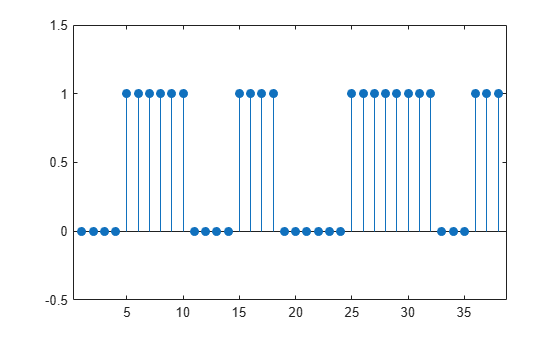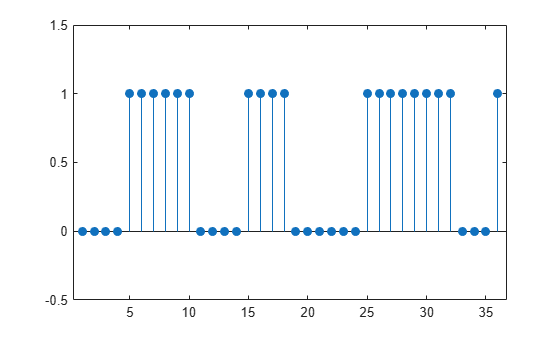Convert matrix of ROI limits to binary mask

Description

example

mask = sigroi2binmask(roilims) converts roilims, a matrix of signal region-of-interest (ROI) limits, to a binary sequence, mask, with true values indicating samples that belong to regions of interest.

example

Examples

collapse all

Consider a two-column matrix of beginning and end samples of four possible regions of interest of a signal. Convert the ROI limits to a logical sequence and display the sequence.

roilims = [5 10; 15 18; 25 32; 36 38];

ylim([0 2]-0.5)Specify the length of the output sequence as 48. sigroi2binmask pads the sequence with false values.

ylim([0 2]-0.5)Specify the length of the output sequence as 36. sigroi2binmask ignores samples beyond the specified sequence length.

ylim([0 2]-0.5)Input Arguments

collapse all

Region-of-interest limits, specified as a two-column matrix of positive integers. The ith row of roilims contains nondecreasing indices corresponding to the beginning and end samples of the ith region of interest of a signal.

Example: [5 8; 12 20; 18 25] specifies a two-column region-of-interest matrix with three regions.

Data Types: single | double | int8 | int16 | int32 | int64 | uint8 | uint16 | uint32 | uint64

Output sequence length, specified as an integer scalar. Regions with indices larger than len are ignored or truncated. If len is greater than max(roilims(:,2)), then sigroi2binmask pads mask with false values.

Data Types: single | double | int8 | int16 | int32 | int64 | uint8 | uint16 | uint32 | uint64

Output Arguments

collapse all

Binary mask, returned as a logical vector with true values indicating samples that belong to a region of interest.